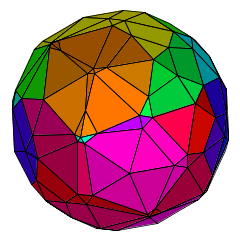Slice draws 3-dimensional slices through the regular 4-dimensional polytopes, as they rotate. By clicking on the applet, you can view each of the six regular polytopes. These are:

• the 4-simplex, with 5 tetrahedral hyperfaces
• the cross-polytope, with 16 tetrahedral hyperfaces
• the hypercube, with 8 cubic hyperfaces
• the 24-cell, with 24 octahedral hyperfaces
• the 600-cell, with 600 tetrahedral hyperfaces
• the 120-cell, with 120 dodecahedral hyperfaces.

In some cases, so long as the slice is generic (that is, it lacks any special alignment with the polytope’s features), the number of faces with various numbers of sides in the polyhedron obtained will be the same:

• for the cross-polytope, there are 8 triangles and 6 quadrilaterals (an irregular cuboctahedron);
• for the 24-cell, there are 6 quadrilaterals and 12 hexagons (an irregular chamfered cube);
• for the 600-cell, there are 152 triangles and 66 quadrilaterals.

In other cases, there are multiple possibilities:

• for the 4-simplex, there can be 4 triangular faces (an irregular tetrahedron), or 2 triangles and 3 quadrilaterals (an irregular triangular prism);
• for the hypercube, there can be 6 quadrilateral faces (an irregular cube), or 2 triangles and 6 pentagons, or 6 quadrilaterals and 2 hexagons;
• for the 120-cell, there are at least 152 distinct possibilities! The least complicated of these is an irregular chamfered dodecahedron, with 12 pentagonal faces and 30 hexagonal faces. This is not the most common form, though.Applets Gallery / Slice / created Monday, 11 March 2002